xGravitational time dilationEncyclopedia
Gravitational time dilation is the effect of time passing at different rates in regions of different gravitational potential; the lower the gravitational potential, the more slowly time passes. Albert Einstein
Albert Einstein
Albert Einstein was a German-born theoretical physicist who developed the theory of general relativity, effecting a revolution in physics. For this achievement, Einstein is often regarded as the father of modern physics and one of the most prolific intellects in human history...

originally predicted this effect in his theory of relativity
Theory of relativity
The theory of relativity, or simply relativity, encompasses two theories of Albert Einstein: special relativity and general relativity. However, the word relativity is sometimes used in reference to Galilean invariance....

and it has since been confirmed by tests of general relativity
Tests of general relativity
At its introduction in 1915, the general theory of relativity did not have a solid empirical foundation. It was known that it correctly accounted for the "anomalous" precession of the perihelion of Mercury and on philosophical grounds it was considered satisfying that it was able to unify Newton's...

.

This has been demonstrated by noting that atomic clock
Atomic clock
An atomic clock is a clock that uses an electronic transition frequency in the microwave, optical, or ultraviolet region of the electromagnetic spectrum of atoms as a frequency standard for its timekeeping element...

s at differing altitudes (and thus different gravitational potential) will eventually show different times. The effects detected in such experiments are extremely small, with differences being measured in nanosecond
Nanosecond
A nanosecond is one billionth of a second . One nanosecond is to one second as one second is to 31.7 years.The word nanosecond is formed by the prefix nano and the unit second. Its symbol is ns....

s.

Gravitational time dilation was first described by Albert Einstein in 1907 as a consequence of special relativity
Special relativity
Special relativity is the physical theory of measurement in an inertial frame of reference proposed in 1905 by Albert Einstein in the paper "On the Electrodynamics of Moving Bodies".It generalizes Galileo's...

in accelerated frames of reference. In general relativity
General relativity
General relativity or the general theory of relativity is the geometric theory of gravitation published by Albert Einstein in 1916. It is the current description of gravitation in modern physics...

, it is considered to be a difference in the passage of proper time
Proper time
In relativity, proper time is the elapsed time between two events as measured by a clock that passes through both events. The proper time depends not only on the events but also on the motion of the clock between the events. An accelerated clock will measure a smaller elapsed time between two...

at different positions as described by a metric tensor
Metric tensor (general relativity)
In general relativity, the metric tensor is the fundamental object of study. It may loosely be thought of as a generalization of the gravitational field familiar from Newtonian gravitation...

of spacetime. The existence of gravitational time dilation was first confirmed directly by the Pound–Rebka experiment.

## Definition

Clock
Clock
A clock is an instrument used to indicate, keep, and co-ordinate time. The word clock is derived ultimately from the Celtic words clagan and clocca meaning "bell". A silent instrument missing such a mechanism has traditionally been known as a timepiece...

s which are far from massive bodies (or at higher gravitational potentials) run faster, and clocks close to massive bodies (or at lower gravitational potentials) run slower (slow is low). This is because gravitational time dilation is manifested in accelerated frames of reference
Frames of Reference
Frames of Reference is a 1960 educational film by Physical Sciences Study Committee.The film was made to be shown in high school physics courses. In the film University of Toronto physics professors Patterson Hume and Donald Ivey explain the distinction between inertial and nonintertial frames of...

or, by virtue of the equivalence principle
Equivalence principle
In the physics of general relativity, the equivalence principle is any of several related concepts dealing with the equivalence of gravitational and inertial mass, and to Albert Einstein's assertion that the gravitational "force" as experienced locally while standing on a massive body is actually...

, in the gravitational field of massive objects.

It can also be manifested by any other kind of accelerated reference frame such as an accelerating dragster
Drag racing
Drag racing is a competition in which specially prepared automobiles or motorcycles compete two at a time to be the first to cross a set finish line, from a standing start, in a straight line, over a measured distance, most commonly a ¼-mile straight track....

or space shuttle
Space Shuttle
The Space Shuttle was a manned orbital rocket and spacecraft system operated by NASA on 135 missions from 1981 to 2011. The system combined rocket launch, orbital spacecraft, and re-entry spaceplane with modular add-ons...

. Spinning objects such as merry-go-rounds and ferris wheel
Ferris wheel
A Ferris wheel is a nonbuilding structure consisting of a rotating upright wheel with passenger cars attached to the rim in such a way that as the wheel turns, the cars are kept upright, usually by gravity.Some of the largest and most modern Ferris wheels have cars mounted on...

s are subjected to gravitational time dilation as an effect of their angular momentum
Angular momentum
In physics, angular momentum, moment of momentum, or rotational momentum is a conserved vector quantity that can be used to describe the overall state of a physical system...

.

This is supported by the general theory of relativity due to the equivalence principle
Equivalence principle
In the physics of general relativity, the equivalence principle is any of several related concepts dealing with the equivalence of gravitational and inertial mass, and to Albert Einstein's assertion that the gravitational "force" as experienced locally while standing on a massive body is actually...

that states that all accelerated reference frames are physically equivalent to a gravitational field of the same strength. For example, a person standing on the surface of the earth experiences exactly the same effect as a person standing in a space ship accelerating at 9.8 m/sec2 (that is, generating a force of 9.8 N/kg, equal to the gravitational field strength of Earth at its surface). According to general relativity, inertial mass and gravitational mass are the same. Not all gravitational fields are "curved" or "spherical"; some are flat as in the case of an accelerating dragster
Dragster
Dragster may refer to*Dragster, a rock band of the New Wave of British Heavy Metal era.*Dragster, a video game released in 1980.*Dragster, a term referring to a custom-built vehicle used in drag racing....

or spacecraft
Spacecraft
A spacecraft or spaceship is a craft or machine designed for spaceflight. Spacecraft are used for a variety of purposes, including communications, earth observation, meteorology, navigation, planetary exploration and transportation of humans and cargo....

. Any kind of g-load
G-force
The g-force associated with an object is its acceleration relative to free-fall. This acceleration experienced by an object is due to the vector sum of non-gravitational forces acting on an object free to move. The accelerations that are not produced by gravity are termed proper accelerations, and...

contributes to gravitational time dilation.
• In an accelerated box, the equation with respect to an arbitrary base observer is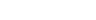, where
•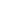is the total time dilation at a distant position,
•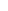is the acceleration of the box as measured by the base observer, and
•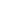is the "vertical" distance between the observers.
When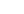is much smaller than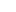, the linear "weak field" approximation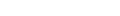may also be used.
• On a rotating disk when the base observer is located at the center of the disk and co-rotating with it (which makes their view of spacetime non-inertial), the equation is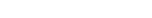, where
•is the distance from the center of the disk (which is the location of the base observer), and
•is the angular velocity of the disk.
(It is no accident that in an inertial frame of reference this becomes the familiar velocity time dilation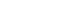).

## Outside a non-rotating sphere

A common equation used to determine gravitational time dilation is derived from the Schwarzschild metric
Schwarzschild metric
In Einstein's theory of general relativity, the Schwarzschild solution describes the gravitational field outside a spherical, uncharged, non-rotating mass such as a star, planet, or black hole. It is also a good approximation to the gravitational field of a slowly rotating body like the Earth or...

, which describes spacetime
Spacetime
In physics, spacetime is any mathematical model that combines space and time into a single continuum. Spacetime is usually interpreted with space as being three-dimensional and time playing the role of a fourth dimension that is of a different sort from the spatial dimensions...

in the vicinity of a non-rotating massive spherically-symmetric object. The equation is: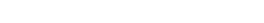, where
•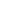is the proper time
Proper time
In relativity, proper time is the elapsed time between two events as measured by a clock that passes through both events. The proper time depends not only on the events but also on the motion of the clock between the events. An accelerated clock will measure a smaller elapsed time between two...

between events A and B for a slow-ticking observer within the gravitational field,
•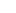is the coordinate time between events A and B for a fast-ticking observer at an arbitrarily large distance from the massive object (this assumes the fast-ticking observer is using Schwarzschild coordinates
Schwarzschild coordinates
In the theory of Lorentzian manifolds, spherically symmetric spacetimes admit a family of nested round spheres. In such a spacetime, a particularly important kind of coordinate chart is the Schwarzschild chart, a kind of polar spherical coordinate chart on a static and spherically symmetric...

, a coordinate system where a clock at infinite distance from the massive sphere would tick at one second per second of coordinate time, while closer clocks would tick at less than that rate),
•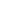is the gravitational constant
Gravitational constant
The gravitational constant, denoted G, is an empirical physical constant involved in the calculation of the gravitational attraction between objects with mass. It appears in Newton's law of universal gravitation and in Einstein's theory of general relativity. It is also known as the universal...

,
•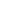is the mass
Mass
Mass can be defined as a quantitive measure of the resistance an object has to change in its velocity.In physics, mass commonly refers to any of the following three properties of matter, which have been shown experimentally to be equivalent:...

of the object creating the gravitational field,
•is the radial coordinate of the observer (which is analogous to the classical distance from the center of the object, but is actually a Schwarzschild coordinate
Schwarzschild coordinates
In the theory of Lorentzian manifolds, spherically symmetric spacetimes admit a family of nested round spheres. In such a spacetime, a particularly important kind of coordinate chart is the Schwarzschild chart, a kind of polar spherical coordinate chart on a static and spherically symmetric...

),
•is the speed of light
Speed of light
The speed of light in vacuum, usually denoted by c, is a physical constant important in many areas of physics. Its value is 299,792,458 metres per second, a figure that is exact since the length of the metre is defined from this constant and the international standard for time...

, and
•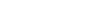is the Schwarzschild radius
The Schwarzschild radius is the distance from the center of an object such that, if all the mass of the object were compressed within that sphere, the escape speed from the surface would equal the speed of light...

of M. If a mass collapses so that its surface lies at less than this radial coordinate (or in other words covers an area of less than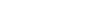), then the object exists within a black hole
Black hole
A black hole is a region of spacetime from which nothing, not even light, can escape. The theory of general relativity predicts that a sufficiently compact mass will deform spacetime to form a black hole. Around a black hole there is a mathematically defined surface called an event horizon that...

.

## Circular orbits

In the Schwarzschild metric, free-falling objects can be in circular orbits if the orbital radius is larger than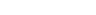. The formula for a clock at rest is given above; for a clock in a circular orbit, the formula is instead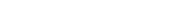## Important things to stress

• According to General Relativity, gravitational time dilation is copresent with the existence of an accelerated reference frame.

• The speed of light in a locale is always equal to c according to the observer who is there. The stationary observer's perspective corresponds to the local proper time
Proper time
In relativity, proper time is the elapsed time between two events as measured by a clock that passes through both events. The proper time depends not only on the events but also on the motion of the clock between the events. An accelerated clock will measure a smaller elapsed time between two...

. Every infinitesimal region of space time may have its own proper time
Proper time
In relativity, proper time is the elapsed time between two events as measured by a clock that passes through both events. The proper time depends not only on the events but also on the motion of the clock between the events. An accelerated clock will measure a smaller elapsed time between two...

that corresponds to the gravitational time dilation there, where electromagnetic radiation and matter may be equally affected, since they are made of the same essence (as shown in many tests involving the famous equation E=mc2). Such regions are significant whether or not they are occupied by an observer. A time delay is measured for signals that bend near the sun, headed towards Venus, and bounce back to earth along a more or less similar path. There is no violation of the speed of light in this sense, as long as an observer is forced to observe only the photons which intercept the observing faculties and not the ones that go passing by in the depths of more (or even less) gravitational time dilation.

• If a distant observer is able to track the light in a remote, distant locale which intercepts a time dilated observer nearer to a more massive body, he sees that both the distant light and that distant time dilated observer have a slower proper time clock than other light which is coming nearby him, which intercept him, at c, like all other light he really can observe. When the other, distant light intercepts the distant observer, it will come at c from the distant observer's perspective.

## Experimental confirmation

Gravitational time dilation has been experimentally measured using atomic clock
Atomic clock
An atomic clock is a clock that uses an electronic transition frequency in the microwave, optical, or ultraviolet region of the electromagnetic spectrum of atoms as a frequency standard for its timekeeping element...

s on airplanes. The clocks aboard the airplanes were slightly faster with respect to clocks on the ground. The effect is significant enough that the Global Positioning System's
Global Positioning System
The Global Positioning System is a space-based global navigation satellite system that provides location and time information in all weather, anywhere on or near the Earth, where there is an unobstructed line of sight to four or more GPS satellites...

artificial satellites
Satellite
In the context of spaceflight, a satellite is an object which has been placed into orbit by human endeavour. Such objects are sometimes called artificial satellites to distinguish them from natural satellites such as the Moon....

need to have their clocks corrected.

Gravitational time dilation has also been confirmed by the Pound–Rebka experiment, observations of the spectra of the white dwarf
White dwarf
A white dwarf, also called a degenerate dwarf, is a small star composed mostly of electron-degenerate matter. They are very dense; a white dwarf's mass is comparable to that of the Sun and its volume is comparable to that of the Earth. Its faint luminosity comes from the emission of stored...

Sirius B and experiments with time signals sent to and from Viking 1
Viking 1
Viking 1 was the first of two spacecraft sent to Mars as part of NASA's Viking program. It was the first spacecraft to successfully land on Mars and perform its mission, and until May 19, 2010 held the record for the second longest Mars surface mission of 6 years and 116 days .- Mission :Following...

Mars lander.